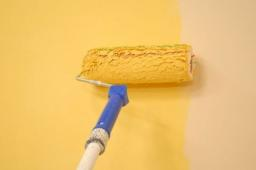# Together 3491

One painter would paint the school in 15 days. Together with the second painter, they painted the school in 6 days. In how many days the second painter would have painted the school himself?

t =  10 d

### Step-by-step explanation:

6·(t/15+1)=t

9t = 90

t = 90/9 = 10

t = 10

Our simple equation calculator calculates it.Did you find an error or inaccuracy? Feel free to write us. Thank you!

Tips for related online calculators
Looking for calculator of harmonic mean?
Looking for a statistical calculator?
Do you have a linear equation or system of equations and looking for its solution? Or do you have a quadratic equation?
Do you want to convert time units like minutes to seconds?